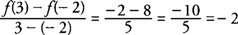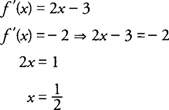## Mean Value Theorem

The Mean Value Theorem establishes a relationship between the slope of a tangent line to a curve and the secant line through points on a curve at the endpoints of an interval. The theorem is stated as follows.

If a function f(x) is continuous on a closed interval [a,b] and differentiable on an open interval (a,b), then at least one number c ∈ (a,b) exists such thatFigure 1 The Mean Value Theorem.

Geometrically, this means that the slope of the tangent line will be equal to the slope of the secant line through (a,f(a)) and (b,f(b)) for at least one point on the curve between the two endpoints. Note that for the special case where f(a) = f(b), the theorem guarantees at least one critical point, where f(c) = 0 on the open interval ( a, b).

Example 1: Verify the conclusion of the Mean Value Theorem for f(x)= x 2−3 x−2 on [−2,3].

The function is continuous on [−2,3] and differentiable on (−2,3). The slope of the secant line through the endpoint values isThe slope of the tangent line isBecause ½ ∈ [−2,3], the c value referred to in the conclusion of the Mean Value Theorem is c = ½